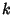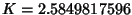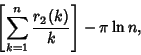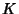## Sierpinski ConstantLet r(n) denote the number of representations ofbysquares, then the Summatory Function ofhas the Asymptotic expansionwhereis the Sierpinski constant. The above plot showswith the value ofindicated as the solid horizontal line.